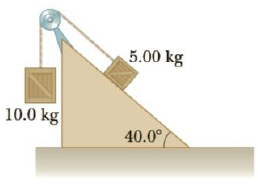Chapter 4, Problem 60P

Chapter
Section
Textbook Problem

Two packing crates of masses 10.0 kg and 5.00 kg are connected by a light string that passes over a frictionless pulley as in Figure P4.60. The 5.00-kg crate lies on a smooth incline of angle 40.0°. Find (a) the acceleration of the 5.00-kg crate and (b) the tension in the string.Figure P4.60

(a)

To determine
The acceleration of the 5.00 kg crate.

Explanation

Given Info: Masses of the crated are m1 =10.0 kg and m2 =5.00 kg. Angle of inclination is 40ο .

The free body diagram is given below.

From the diagram,

Tm2gsin40ο=m2a (I)

m1gT=m1a (II)

• T is the tension.
• m1 and m2 are the masses.
• g is the acceleration due to gravity.
• a is the acceleration.

Add Equations (I) and (II).

g(m1m2sin40ο)=a(m1+m2)

On Re-arranging, the acceleration is,

a=g(m1m2sin40οm1+m2)

Substitute 9

(b)

To determine
The tension in the string.

Still sussing out bartleby?

Check out a sample textbook solution.

See a sample solution

The Solution to Your Study Problems

Bartleby provides explanations to thousands of textbook problems written by our experts, many with advanced degrees!

Get Started